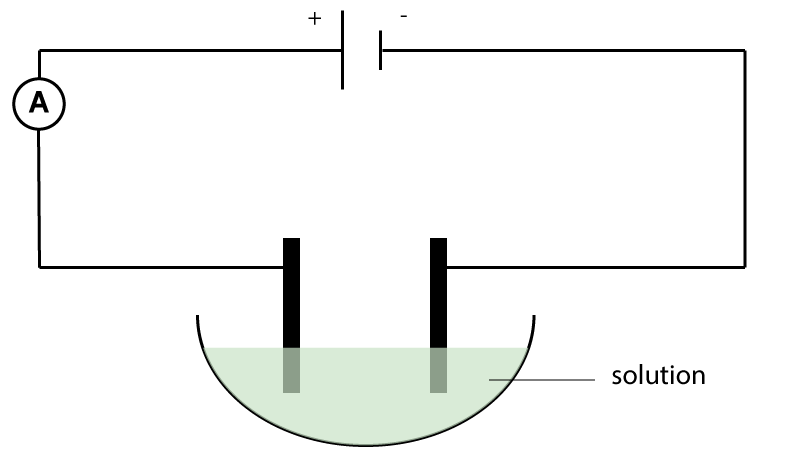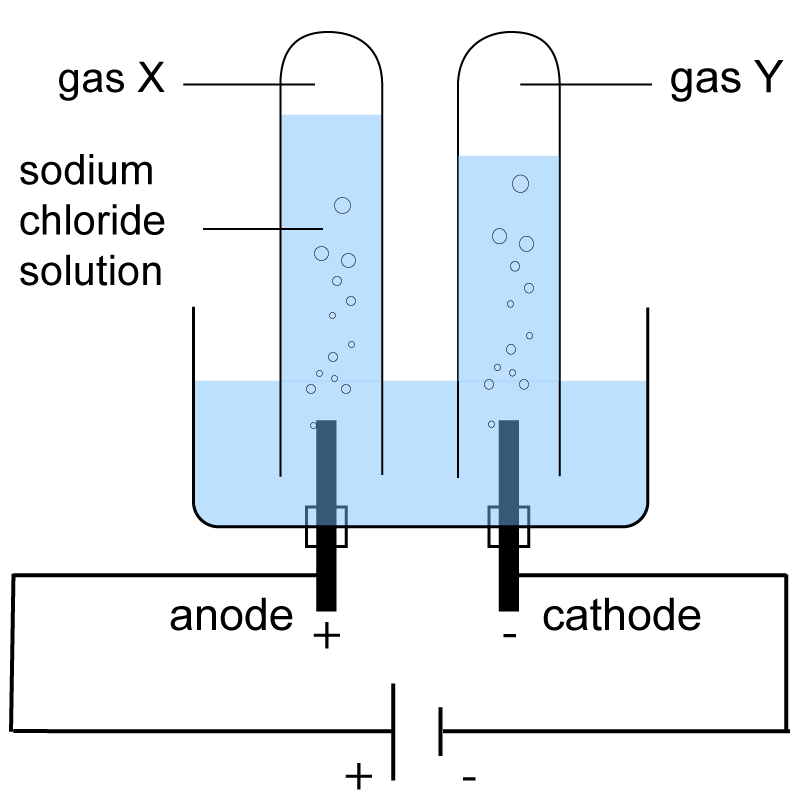10 minutes maximum! Can you do it in 5?

1. The products at the electrodes in the electrolysis of molten potassium iodide are:

 Product at negative electrode Product at positive electrode A potassium iodine B potassium iodide C iodine potassium D iodide potassium

Q2-5. Electrolysis of molten lead bromide is carried out using the following circuit:2. The current is carried around the circuit by:

 In molten lead bromide In the wires A electrons ions B electrons electrons C ions ions D ions electrons
3. The bulb will not light when lead bromide is solid because ...
• A. solid lead bromide is covalently bonded
• B. solid lead bromide is too tightly packed for electrons to get through
• C. the ions in solid lead bromide are not free to move around
• D. the atoms in solid lead bromide are not free to move around

4. The balanced half-equation and the type of reaction occurring at the negative electrode is:

 Half-equation at negative electrode Type of reaction A Pb2+ → Pb + 2e- reduction B Pb2+ → Pb + 2e- oxidation C Pb2+ + 2e- → Pb reduction D Pb2+ + 2e- → Pb oxidation

5. The balanced half-equation and the type of reaction occurring at the positive electrode is:

 Half-equation at positive electrode Type of reaction A 2Br- + 2e-  → Br2 reduction B 2Br- → Br2  +  2e- oxidation C Br- → Br  +  e- oxidation D Br-  +  e-  →  Br reduction

Q6-7.   Four substances were dissolved in water and connected in turn into the circuit below.The results are shown in the table:

 Current /A Product at cathode (-) Product at anode (+) A 0.6 copper chlorine B 0.6 copper oxygen C 1.1 hydrogen oxygen D 0.9 hydrogen chlorine

6. Which solution could be sulphuric acid?

7. Which solution could be copper(II)nitrate ?

Q8-9.
The electrolysis of a concentrated solution of sodium chloride produced two gases, X and Y :8. The identity of gas X and a test to confirm its identity is:

 Gas X Test to confirm identity of gas X A chlorine Turns damp blue litmus paper red B chlorine bleaches damp blue litmus paper to white C oxygen Relights a glowing splint D oxygen Ignites with a pop with a burning splint
9. The correct half-equation for the formation of gas Y is:
• A. H+ + e-  H
• B. 2H+ + 2e- H2
• C. 2H+H2 + 2e-
• D. Na+ + e-  Na

10. Aluminium is extracted by the electrolysis of molten aluminium oxide, Al2O3.

 Product at cathode Equation at anode A aluminium 2O2- →  O2 + 4e- B aluminium O2- →  O + 2e- C oxygen Al3+ + 3e- →  Al D oxygen Al3+ →  Al + 3e-